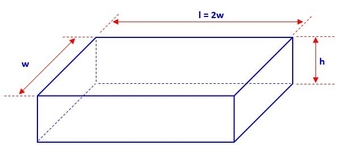# A rectangular storage container with an open top is to have a volume of 10 m^3. The length of the...

## Question:

A rectangular storage container with an open top is to have a volume of 10 {eq}m^3{/eq}. The length of the base is twice the width. Material for the base costs $20 per square meter. Material for the sides costs$12 per square meter. Find the cost of materials for the cheapest such container. (Round your answer to the nearest cent.)

## Application of Differential Calculus: Optimization

According to Second Derivative Test, a function f(x) is said to have a minimum value at {eq}x = n {/eq} if {eq}f'(n) = 0 \text{ and } f''(n) >0. {/eq} The same function is said to have a maximum value at {eq}x = n {/eq} if {eq}f'(n) = 0 \text{ and } f''(n) <0. {/eq} We can use this concept to find the dimension of the box that will cost the cheapest.

The figure below shows an open top rectangular box.First, we need to determine the volume of the rectangular box. Note that the formula for...

Become a Study.com member to unlock this answer! Create your account